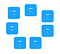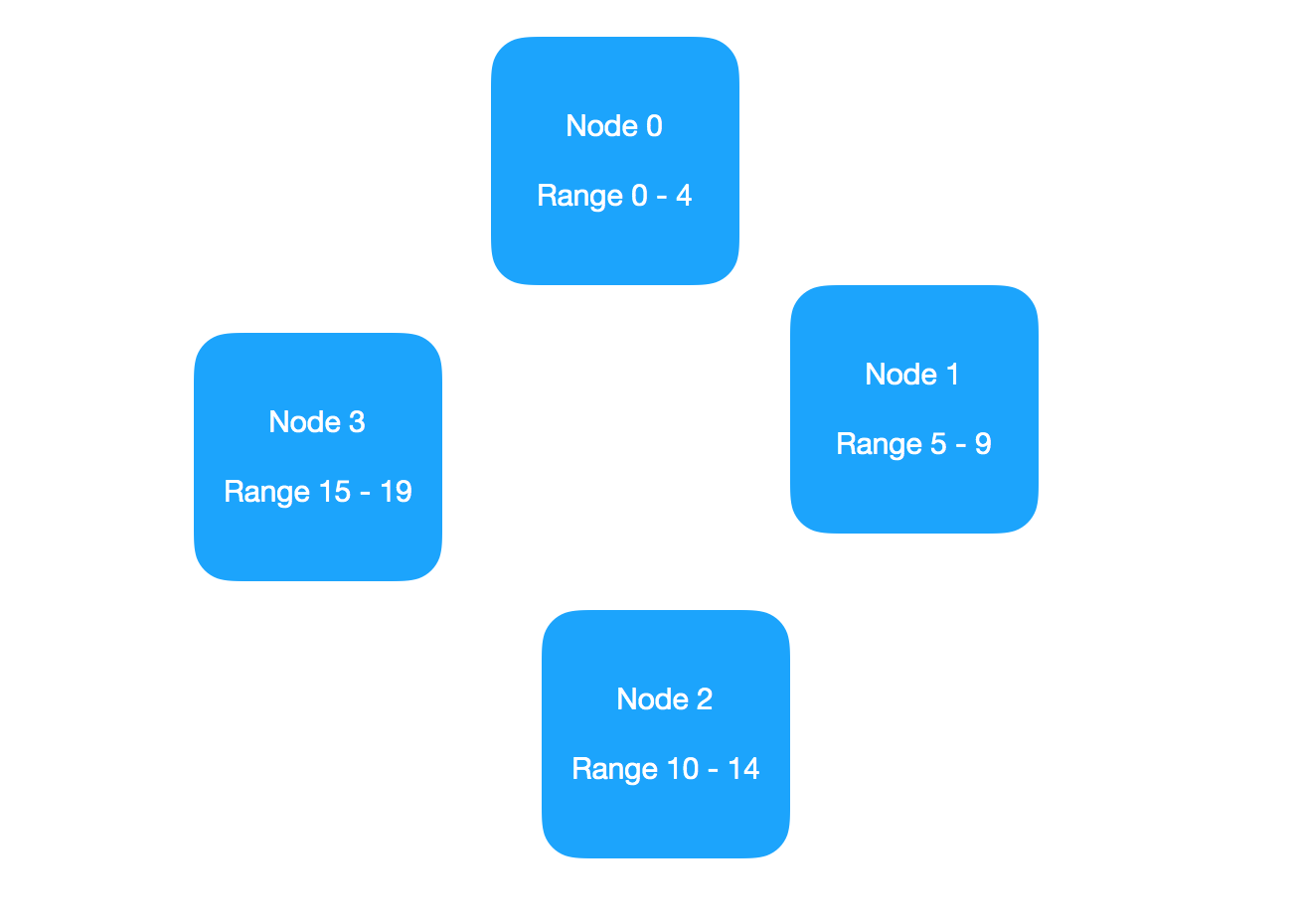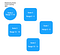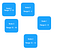# Hash Tables In Distributed System

Requirement

Lets say we have few million of keys along with a value. For example, the order id may have a reference to the order details on an e-commerce website.

We want to have a O(1) lookup for a key. Assuming the keys cannot fit in a single machine (unless we use a very large machine where the cost is not justified) we need a distributed system to store keys.

Solution

Lets say that we have n systems. There could be multiple ways to distribute the keys in these n systems.

Let us number each node as 0……n-1.For N = 7

Hash Function

A hash function is a function

A hash function is called consistent so that

In our case we need a consistent hash function. Let us define our hash function h(x) = x mod R , where R is a number such that R > n

Where n is the total number of nodes.

We see that range of x mod R => 0….R-1

Each node now stores hash values between a rangeWhen R = 20 and n = 4

When we need to find the value against a key K, we calculate h(k) and ask the node corresponding h(k) for the value.

Currently we are not concerned about replication or node addition. A trivial redundancy could be created by having each node also be responsible for secondary range. In case of replication at 3 nodes, each node now has primary, secondary and tertiary ranges. This could be extended till n replication.

For addition of a node, the node joins the group. The range is split from one of the nodes, and the new range is provided to the new node. The node is currently shadowing and will copy all keys data from primary node for the range. Once the copy is complete, the split is broadcasted and the new node processes for new range.Adding a nodeAddition complete

Removing a node is similar, the secondary node takes responsibility of the range when a node is removed / is no longer responding. It becomes the primary owner for the range of hash values.

What happens when there are large number of nodes?

Let us say that the number of nodes in the system increases to a large number. Also, lets say that the client can contact any node and ask for a value. It is the responsibility of the node to figure out the appropriate node for the key, transfer the request to that node.

O(1) Solution

Let each node store the node range and address of the corresponding node. For the input key, h(x) will give the range. If it is this node, get from the map and return, else do a lookup of node address and transfer.

This does the job, but the additional overhead increases when there are a large number of nodes. Also, now each node needs to have updated information of all entries and exit to the cluster.

O(n) Solution

Let each node store information of the previous and next node. If h(x) is not equal to this node, then transfer request to the next node. The next node processes it similarly.

O(log(n)) Solution

We can use the concept of maintaining a finger table (refer https://en.wikipedia.org/wiki/Chord_(peer-to-peer) )

Lets say each node with value i, maintains a fixed size array where it stores

(Lets say that we have n nodes, we store log(n) array)

Index | Value -> Node Address

0 | i+1 -> next node value

1 | i+2 -> …..

2 | i+4 -> ….

i | i+ 2^i-1 -> ……

When the request comes for a key K, say h(K) = V.

If V = i, then this node responds.

Else, request is routed to the node corresponding to value, such that for some index j,

It can now be easily proven that the lookup can be done in O(log(n))

I am passionate about designing and developing complex systems that impact people’s life. A avid reader and a believer of multi-disciplinary teaching.

## More from Jaiprakash

I am passionate about designing and developing complex systems that impact people’s life. A avid reader and a believer of multi-disciplinary teaching.